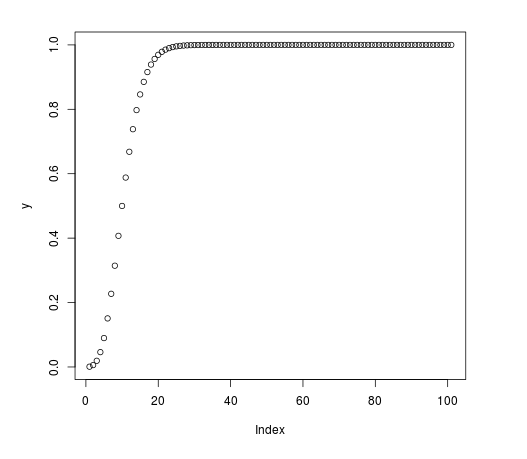Related Articles
Compute the Negative Binomial Cumulative Density in R Programming – pnbinom() Function
• Last Updated : 25 Jun, 2020

`pnbinom()` function in R Language is used to compute the value of negative binomial cumulative density. It also creates a density plot of the negative binomial cumulative distribution.

Syntax: pnbinom(vec, size, prob)

Parameters:
vec: x-values for binomial density
size: Number of trials
prob: Probability

Example 1:

 `# R program to compute``# Negative Binomial cumulative Density`` ` `# Vector of x-values``x <``-` `seq(``0``, ``10``, by ``=` `1``)`` ` `# Calling pnbinom() Function``y <``-` `pnbinom(x, size ``=` `10``, prob ``=` `0.5``)``y`

Output:

```  0.0009765625 0.0058593750 0.0192871094 0.0461425781 0.0897827148
 0.1508789063 0.2272491455 0.3145294189 0.4072647095 0.5000000000
 0.5880985260
```

Example 2:

 `# R program to compute``# Negative Binomial Cumulative Density`` ` `# Vector of x-values``x <``-` `seq(``0``, ``100``, by ``=` `1``)`` ` `# Calling pnbinom() Function``y <``-` `pnbinom(x, size ``=` `10``, prob ``=` `0.5``)`` ` `# Plot a graph``plot(y)`

Output:Attention reader! Don’t stop learning now. Get hold of all the important DSA concepts with the DSA Self Paced Course at a student-friendly price and become industry ready.

My Personal Notes arrow_drop_up